# Test: Coupled Circuits - 3

## 10 Questions MCQ Test Network Theory (Electric Circuits) | Test: Coupled Circuits - 3

Description
Attempt Test: Coupled Circuits - 3 | 10 questions in 30 minutes | Mock test for Electrical Engineering (EE) preparation | Free important questions MCQ to study Network Theory (Electric Circuits) for Electrical Engineering (EE) Exam | Download free PDF with solutions
QUESTION: 1

### Leq =?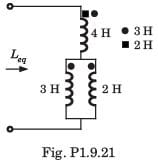Solution:

Let I1 be the current through 4 H inductor and I2 and I3 be the current through 3 H, and 2 H inductor respectively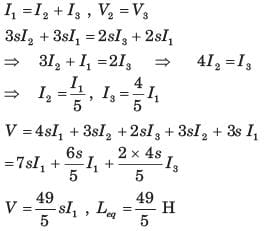QUESTION: 2

### Consider the circuit shown in fig. P1.9.22–24.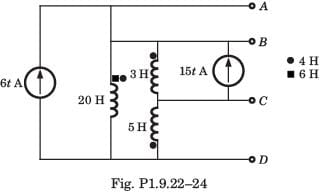Q. The voltage VAG of terminal AD is

Solution: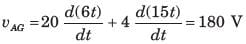QUESTION: 3

### Consider the circuit shown in fig. P1.9.22–24.Q. The voltage vBG of terminal BD is

Solution: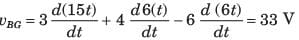QUESTION: 4

Consider the circuit shown in fig. P1.9.22–24.Q. The voltage vCG of terminal CD is

Solution: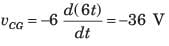QUESTION: 5

In the circuit of fig. P1.9.33 the ω = 2 rad/s. The resonance occurs when C is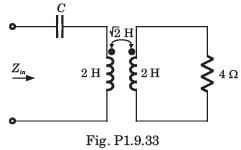Solution: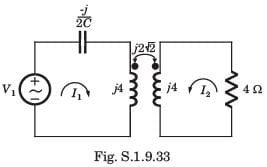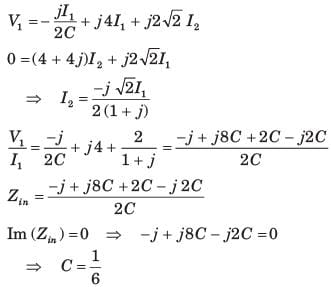QUESTION: 6

In the circuit of fig. P1.9.34, the voltage gain is zero at ω = 333.33 rad/s. The value of C is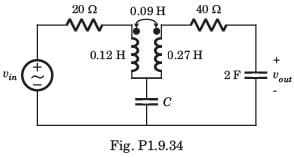Solution: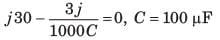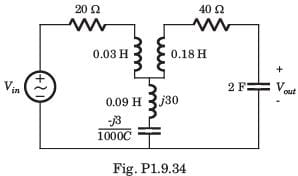QUESTION: 7

In the circuit of fig. P1.9.35 at ω = 333.33 rad/s, the voltage gain vout/vin is zero. The value of C is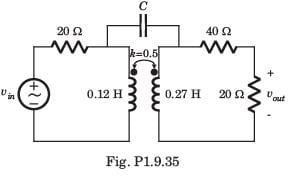Solution:

The π  equivalent circuit of coupled coil is shown in fig. S1.9.35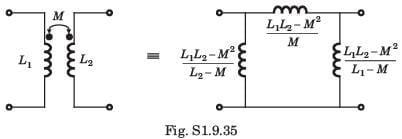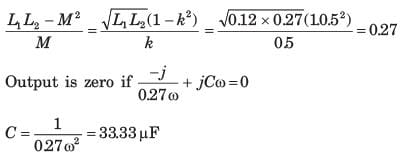QUESTION: 8

The Thevenin equivalent at terminal ab for the network shown in fig. P1.9.36 is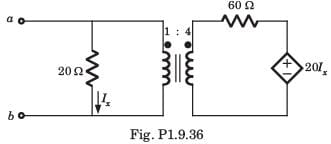Solution:

Applying 1 V test source at ab terminal,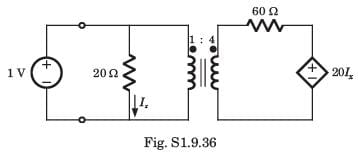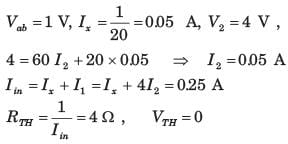QUESTION: 9

In the circuit of fig. P1.9.37 the maximum power delivered to RL is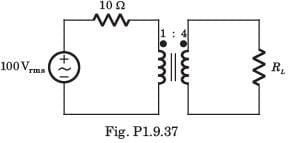Solution:

Impedance seen by RL = 10 x 42 = 160 Ω
For maximum power RL = 160 Ω, Zo = 10Ω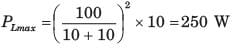QUESTION: 10

The average power delivered to the 8Ω load in the circuit of fig. P1.9.38 is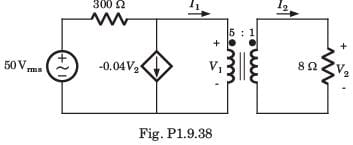Solution: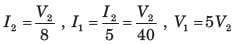Use Code STAYHOME200 and get INR 200 additional OFF Use Coupon Code